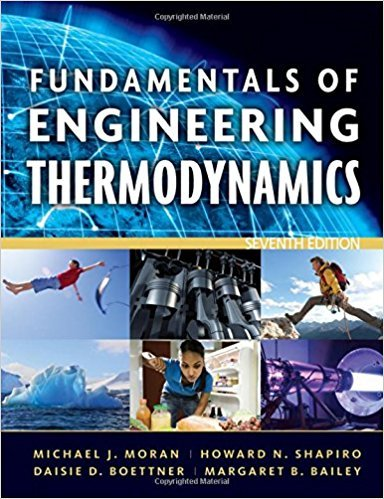×
Get Full Access to Fundamentals Of Engineering Thermodynamics - 7 Edition - Chapter 4 - Problem 19p
Get Full Access to Fundamentals Of Engineering Thermodynamics - 7 Edition - Chapter 4 - Problem 19p

×

# Applying Conservation of MassA water storage tankISBN: 9780470495902 50

## Solution for problem 19P Chapter 4

Fundamentals of Engineering Thermodynamics | 7th Edition

• Textbook Solutions
• 2901 Step-by-step solutions solved by professors and subject experts
• Get 24/7 help from StudySoup virtual teaching assistantsFundamentals of Engineering Thermodynamics | 7th Edition

4 5 1 420 Reviews
13
2
Problem 19P

Problem 19P

Applying Conservation of Mass

A water storage tank initially contains 100,000 gal of water. The average daily usage is 10,000 gal. If water is added to the tank at an average rate of 5000[exp(−t/20)] gallons per day, where t is time in days, for how many days will the tank contain water?

Step-by-Step Solution:
Step 1 of 3

Step 2 of 3

Step 3 of 3

##### ISBN: 9780470495902

The answer to “Applying Conservation of MassA water storage tank initially contains 100,000 gal of water. The average daily usage is 10,000 gal. If water is added to the tank at an average rate of 5000[exp(?t/20)] gallons per day, where t is time in days, for how many days will the tank contain water?” is broken down into a number of easy to follow steps, and 51 words. Fundamentals of Engineering Thermodynamics was written by and is associated to the ISBN: 9780470495902. The full step-by-step solution to problem: 19P from chapter: 4 was answered by , our top Engineering and Tech solution expert on 07/20/17, 09:01AM. Since the solution to 19P from 4 chapter was answered, more than 373 students have viewed the full step-by-step answer. This textbook survival guide was created for the textbook: Fundamentals of Engineering Thermodynamics, edition: 7. This full solution covers the following key subjects: Water, tank, average, gal, days. This expansive textbook survival guide covers 14 chapters, and 1501 solutions.

Unlock Textbook Solution